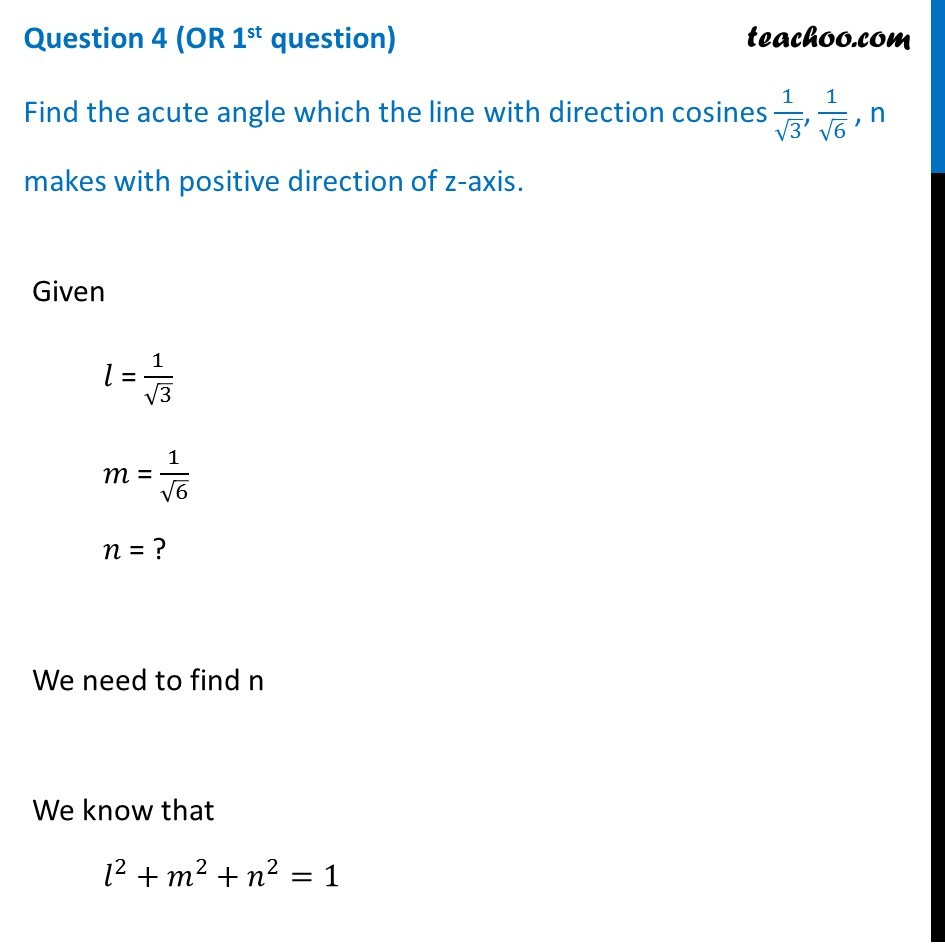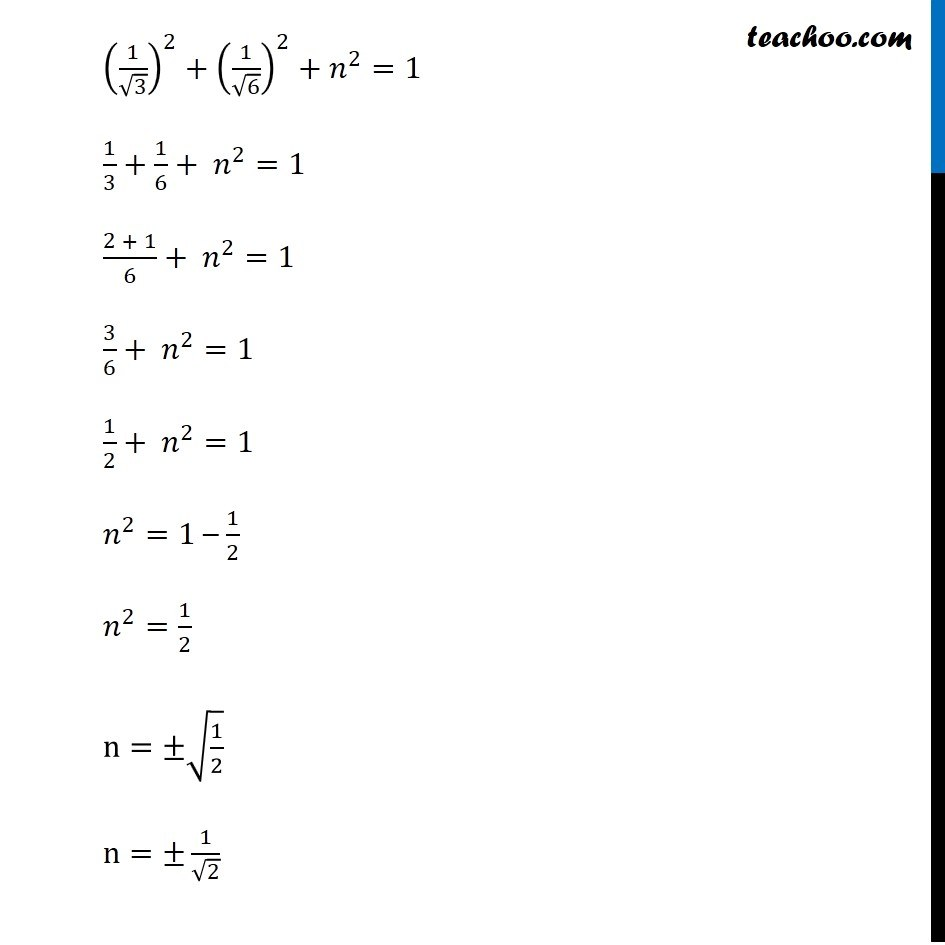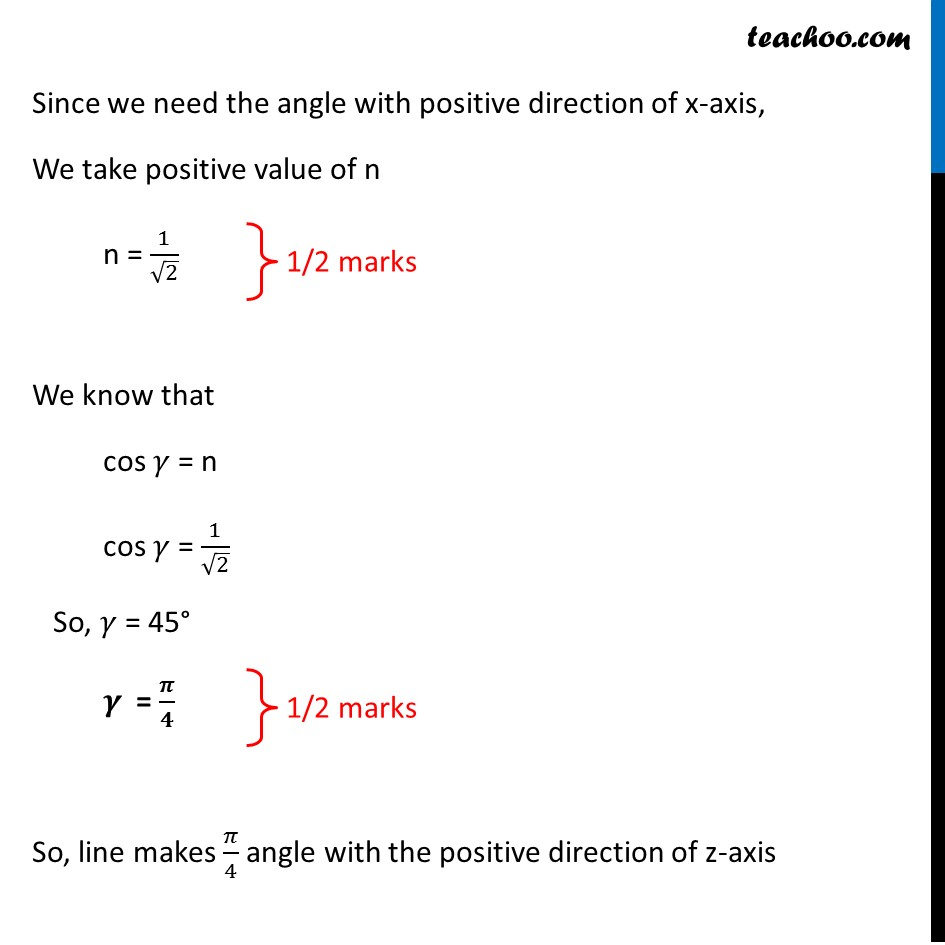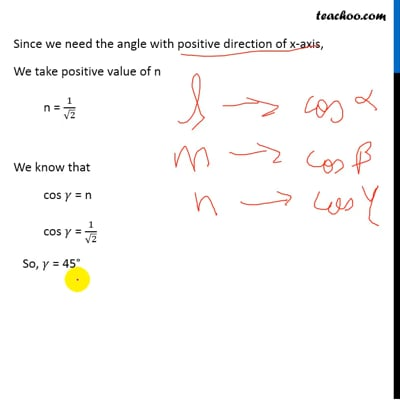CBSE Class 12 Sample Paper for 2019 Boards

Class 12
Solutions of Sample Papers and Past Year Papers - for Class 12 Boards

Question 4 (OR 1 st question)

Find the acute angle which the line with direction cosines 1/√3, 1/√6, n makes with positive direction of z-axis.This video is only available for Teachoo black users

Introducing your new favourite teacher - Teachoo Black, at only ₹83 per month

### Transcript

Question 4 (OR 1st question) Find the acute angle which the line with direction cosines 1/√3, 1/√6 , n makes with positive direction of z-axis. Given 𝑙 = 1/√3 𝑚 = 1/√6 𝑛 = ? We need to find n We know that 𝑙^2+𝑚^2+𝑛^2=1 (1/√3)^2+(1/√6)^2+𝑛^2=1 1/3+1/6+ 𝑛^2=1 (2 + 1)/6+ 𝑛^2=1 3/6+ 𝑛^2=1 1/2+ 𝑛^2=1 𝑛^2=1 – 1/2 𝑛^2=1/2 n=±√(1/2) n=±1/√2 Since we need the angle with positive direction of x-axis, We take positive value of n n = 1/√2 We know that cos 𝛾 = n cos 𝛾 = 1/√2 So, 𝛾 = 45° 𝜸 = 𝝅/𝟒 So, line makes 𝜋/4 angle with the positive direction of z-axis